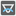## Question related to missionThe Angles of a Triangle

Rounding of an angle value can introduce an error of up to 0.5, so the sum of three rounded angles of a triangle can be 179, 180 or 181 degrees. And the solution with something like

```angleC = 180 – angleA – angleB
```

where angleA and angleB are rounded angle values is wrong! I suggest adding new test cases:

```assert checkio(1, 2, 2) == [29, 76, 76], "sum == 181"
assert checkio(1, 3, 3) == [19, 80, 80], "sum == 179"
```

and rechecking.